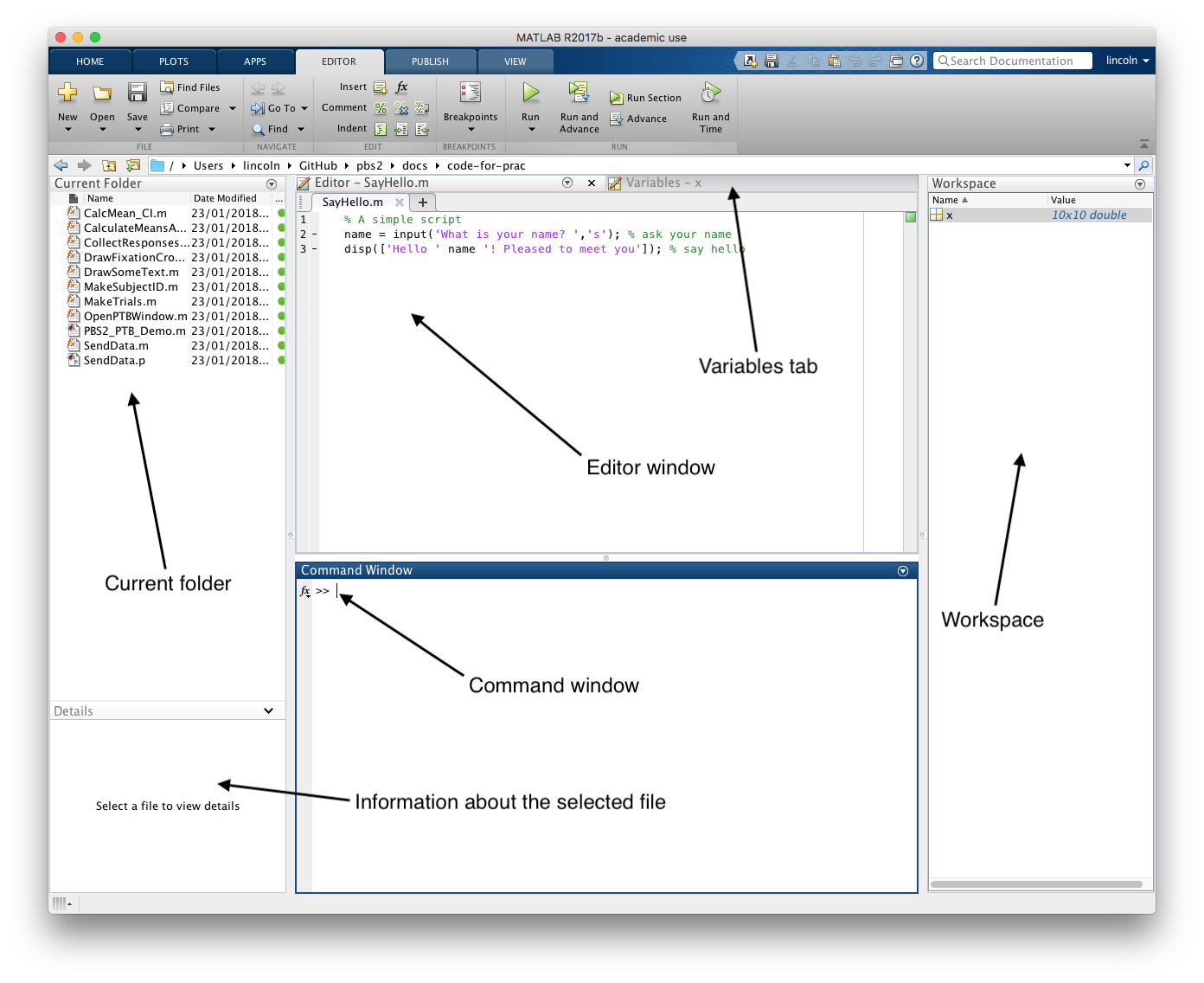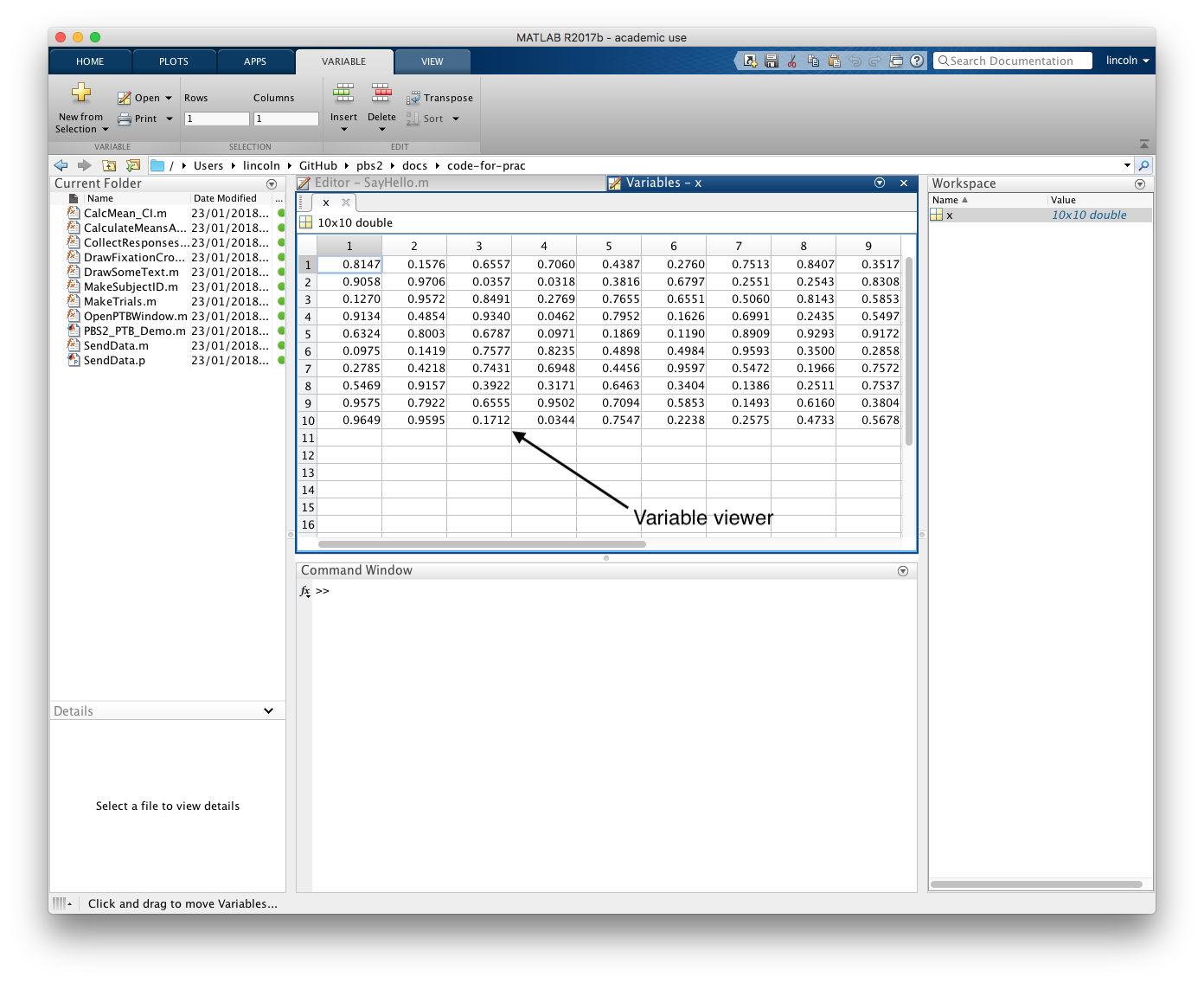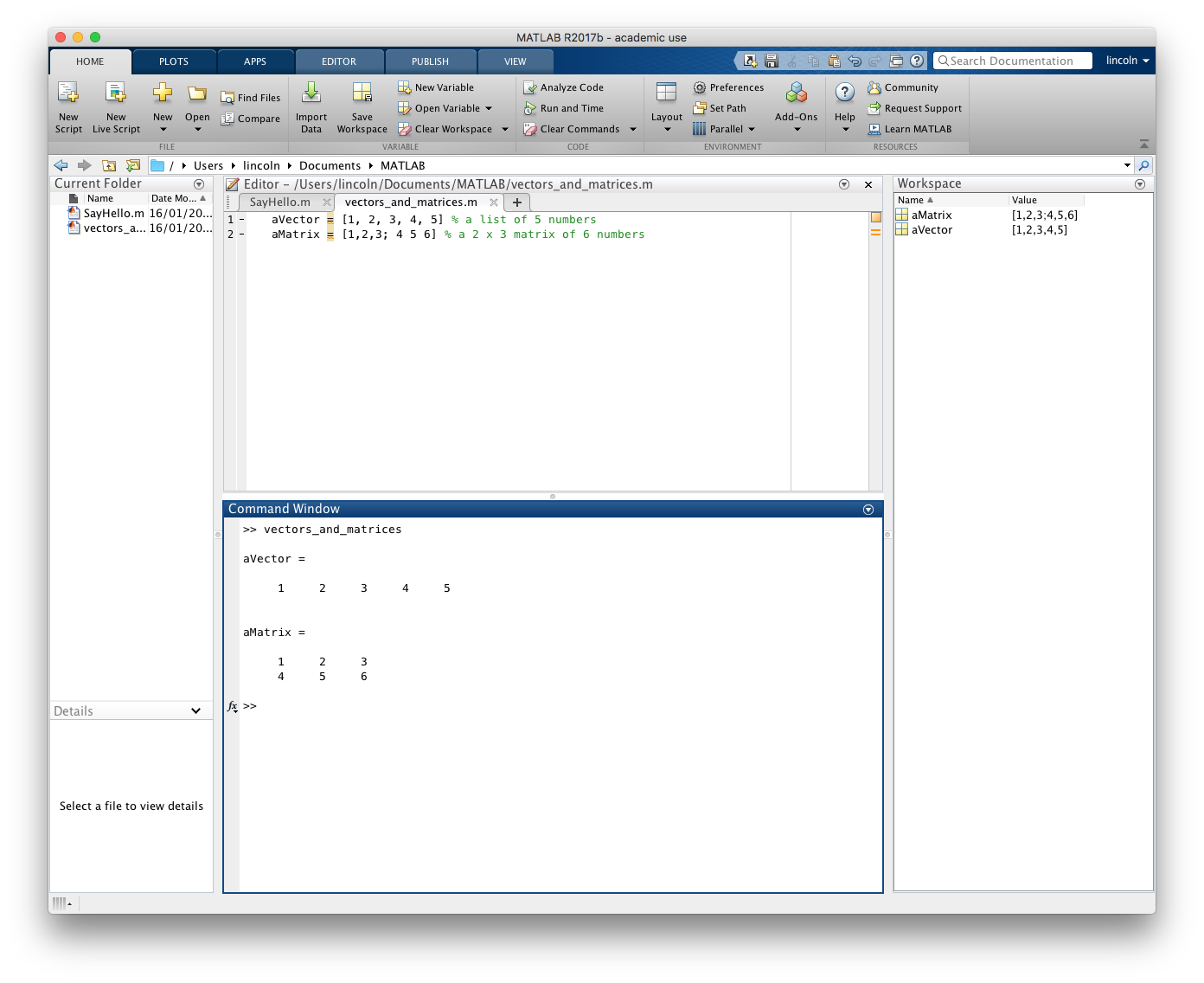Back to Main Page

## Introduction to scientific computing with Matlab and PsychToolBox

Dr Lincoln Colling

ljc65@cam.ac.uk

http://pbs2.mindsci.net

Lecture 1

## Course website

The website `http://pbs2.mindsci.net` will contain a lot of information that will be useful for this course, including:

1. The lecture slides (with clickable links!)
2. Sample code snippets
3. Cheat sheets and tips

Make sure that you visit it!

## What is MATLAB?

• `Matlab` is a high-level programming language
• While not as flexible as some traditional languages it's generally a lot quicker and easier to write advanced programs in `Matlab`
• Suited for many tasks in Psychology research e.g., signal processing (EEG research), image processing (vision research), modelling (psychophysics and signal detection), and more

Note: When referring to programming languages 'high-level' means that there is a high level of abstraction from the instruction set the computer is actually using. Low-level means that you're using a language that is close to the computers native instruction set. High-level languages look more like natural human language.

## The aim of this lecture series

• Get you familiar with the basics of programming
• Get you familiar with how to find help when you encounter a new programming challenge
• Introduce you to the basics of `PsychToolBox`, a set of `Matlab` tools for stimulus presentation and response collection

## Why MATLAB and not...

`Python`, `R`, `Julia`, `C#`, `perl`, or ...

• `Matlab` provides a one-stop-shop will all the common tools you'll need for research in psychology
• Although focused on `Matlab` you'll hopefully learn enough of the basics so that when you encounter other languages you'll be able to translate what you know from `Matlab` into these new languages.

Some fans of Free and Open Source Software don't like `Matlab` because it is expensive proprietary software. But `Matlab` syntax is almost completely compatible with `Octave`, a FOSS alternative to `Matlab`

Note: Learning new languages is relatively easy once you understand the basics of one. I personally use several languages in my day-to-day work (including `R`, `Matlab`, `Python`, and `perl`). Each of these are equally capable, but sometimes one language might be better suited to one job relative to the other.

## My philosophy of programming

• Often people don't care how they solve the problem, only that their code works...

• But it's better to do it right the first time and write code with 3 assumptions in mind
1. That you'll understand nothing next time you look at the code
2. That somebody else will be using your code
3. That your code will need to run on another computer at some point

## The process of writing programs

• Involves breaking down to the problem into solvable steps

• Lots of trial-and-error to check how bits of the code work

• Debugging and finding errors can often take longer than the initial programming

• The more you practice the better you'll get!

Note: If you're looking for some programming challenges that will help you practice your problem solving skills then check out Project Euler (projecteuler.net).

### Knowing how/where to get help

Don't worry if you can't remember all the syntax and commands or can't figure out a problem

• You don't need to remember something if you know how to look it up

• If you're having trouble solving a problem chances are somebody has encountered a similar problem, so know how to look for other people's solutions. Google, Stack Overflow, and the Matlab Central websites are your friend!

Note: The difference between asking a good question and a bad question is that a good question will provide enough information so that the person helping you will be able to give you a helpful answer. Before asking a question on Stack Overflow or Matlab Central I recommend reading some tips on how to ask a good question. Check out Jon Skeey’s coding blog and Stack Overflow to get tips on how to ask a good question.

## Getting started

You can install `Matlab` on your own computer.

### Steps

1. Get a free licence key from UIS
2. Create an account on the Mathworks website
3. Associate your licence key with you Mathworks account
4. Download and install `Matlab` using the online activation option

Note: Matlab is available for Windows, MacOS, Linux. If you're using the something like a chromebook, then you can use matlab online.

## The basics of Matlab

### The Matlab windowNote: The `Matlab` window has many parts. These include a list of the files in your Current Folder, the list of variables in your Workspace, an Editor/Variables editor where you can read and edit scripts, and a Command Window for typing in commands that are run immediately.

The Command Window allows you to type in and execute `Matlab` commands directly (just as if they were in a script).

Any messages displayed by `Matlab` (such as error or warning messages) or messages displayed by your code are shown in the Command Window.

The Editor window is where you can edit scripts and functions.

You can also highlight and right-click/CTRL-click to run specific chunks of code. This is useful for debugging your code (that is, finding out what is causing a problem).

The Workspace provides a list of the variables currently in memory.

These variables are accessible to all scripts that you run.

Each function has it’s own workspace, but it won’t be shown in the Workspace window unless you pause the execution of the function (we’ll return to this later when we talk about Scope).

The Variables editor shows you the contents of a variable.

You can also directly edit values in the Variables editor, but this is generally a very bad idea. It’s better to write some code to edit the values. So you can keep track of the changes you’re making.

The Current Folder list is a list of the files in your current folder.

If any of the files are `Matlab` data files (`*.mat`) or `Matlab` function or script files (`*.m`) then you can open them from this window.

Some other types of files (e.g., `*.csv` and `*.txt` files) can also be opened from this window.

### How to get help

Two commands that you can use at the Command Window to get help.

• the `help` function: Prints out some information in the Command Window

• the `doc` function: Opens up a new window with detailed information usually including examples of the function being used

``````>> help sin
sin    Sine of argument in radians.
sin(X) is the sine of the elements of X.

Reference page for sin
Other functions named sin``````

• Once you've installed `Matlab`, then just start it up by clicking on the icon

• If you can't install `Matlab` on your computer (e.g., if you have a Chromebook or similar) then you can use Matlab Online once you've set up an Mathworks account
• Make sure you're in the folder you want to store your scripts and then type the following command at the prompt

``>> unzip('https://git.io/vNScy')``

Check out the guide on the course website

### Scripts

• Scripts are just collections of commands. You could type these commands into the command prompt but scripts are a good way of keeping them together.
``````% A simple script
disp(['Hello ' name '! Pleased to meet you']); % say hello``````
• Scripts get stored in a file with a `.m` extension e.g., `SayHello.m`.

• You can run a script by typing the name of the script into the command prompt

Note: The names of scripts must start with a letter, and can only contain letters, numbers, and underscores. No spaces, or non-alphanumeric characters are allowed.

# Our first script

``````% A simple script
disp(['Hello ' name '! Pleased to meet you']); % say hello``````

Our first simple script contains a number of parts. These include `variables`, calls to `functions`, and `comments` that tell us what bits of it do.

• `comments` are important because they help other people (and you at a later date) understand your code. They are like little notes in the code

• In `Matlab` comments are marked with `%`. Anything that comes after the `%` isn’t run by `Matlab`

``````A = 1 % This is a comment and isn't run by matlab

% This is also a comment, but it's on a line of it's own``````

## Variables

• `variables` are used for storing information
• They come in many types, which can store different types of information
• You can assign content to a variable with an `=` sign
``````% Store 'Lincoln' in a variable called 'name'
name = 'Lincoln'
% Store '1' in a variable called 'week'
week = 1``````

### Variable types

#### Numbers

• You can store numbers in a `double` or an `integer`.
• A `double` is used for storing decimals, and an `integer` is used for storing whole numbers. In regular use, `matlab` doesn’t require you to do anything different when assigning decimals/whole numbers to variables
``````% assign some numbers to some variable
aDecimal = 1.0 % a double
aWhole = 1 % an integer``````

Note: We’ll discuss some technical issues that arise because of how computers represent numbers later, but for now you don’t need to worry about computers actually represent decimals

##### Vectors and matrices

Sometimes you might want to store multiple numbers

• a `vector` is a list of numbers
``aVector = [1, 2, 3, 4, 5] % a list of 5 numbers``
• a `matrix` is a list of lists
``aMatrix = [1,2,3; 4 5 6] % a 2 x 3 matrix of 6 numbers``
• a `matrix` can be any number of dimensions
• a `matrix` or `vector` can store decimals or whole numbers or a mix of both

Note: When assigning numbers to a `vector` or `matrix` the content goes inside `[` and `]`. You can separate numbers in the same dimension by `,` and you can separate dimensions with `;`##### Filling vectors and matrices

There are lots of ways to fill a `vector` or `matrix` with numbers.

``````aVector = [1 2 3]   % typing out all the numbers
aVector = [1:3] % a sequence of numbers from 1 to 3
aVector = [1:2:5] % 1 to 5 going up in steps of 2
aVector = [3:-1:1] % backwards from 3 to 1
aVector = randperm(4,4) % 4 numbers in random order
aMatrix = zeros(2,3) % a 2 x 3 matrix of 0s
aMatrix = ones(2,2,2) % a 2 x 2 x 2 matrix of 1s
aMatrix = rand(3,2) % a 3 x 2 matrix of random numbers (0—1)``````

#### Getting information about vectors and matrices

• Once you’ve created a `vector` or a `matrix` then you can get information about it’s size with the `size()`, `length()`, and `numel()` functions
``````aMatrix = [1, 2; 3, 4] % create a matrix
size(aMatrix) % returns: [2 2]
numel(aMatrix) % returns: 4
aVector = [1:10] % create a vector
length(aVector) % returns: 10``````
• Knowing how big a `matrix` or a `vector` is can be useful when you want to write loops that operate on each element of the variable

#### Accessing specific elements

• Sometimes you might want to access a specific element. You can do this by specifying its location (or index)
``````aVector = [1 2 3 4 5] % create a vector
aVector(4) % returns: 4
aVector(4) = 7
aVector % returns: [1 2 3 7 5]
aVector(end) % returns 5``````
``````aMatrix = [1, 2; 3, 4] % create a matrix
aMartix(1,1) % returns: 1
aMatrix(1,:) % returns: [1, 2]
aMatrix(:,1) % returns [1; 3]``````

Note: Notice that `matlab` uses `1` for the first element. This is the same as in `R`, but is different to some other programming languages (e.g., `C/C++`) which uses `0` for the first element.

In later lectures we'll talk about how you can find the index (or indices) that corresponds to a specific value.

#### Concatenating vectors and matrices

• Sometimes you might want to join up two matrices or vectors
• To do this, you can use the `horzcat()` and `vertcat()` functions
• These join vectors/matrics horizontally and vertically.
• Be careful, though, because to join two matrices horizontally they must be the same height and to join to vectors vertically they must be the same width

### Strings of letters

• a `string` is like a `vector`, but it contains letters rather than numbers
• You put their content inside `'` instead of `[`/`]`
``````name = 'Lincoln' % define a string
name(1) % returns: 'L'
name(end) % returns: 'n'``````

#### Concatenating strings

• You can join two `string`s just as you would join two `matrix`s or `vector`s
• Because all strings are the same height, you can join them just by putting the parts inside `[`/`]`
``````>> firstName = 'Lincoln'; % define a string
>> lastName = 'Colling'; % define another string
>> [firstName ' ' lastName]

ans =

'Lincoln Colling'

>> ``````

### Cell arrays

• `cell` arrays are incredibly flexible. They allow you to mix and match data types so you can store letters and numbers.
• Each element in a `cell` array is like it’s own variable
• The content of a `cell` goes inside `{`/`}`
• `cell` arrays can be multidimensional like a `matrix`
``````aCellArray = {1 '2' 3 '4' [1 2 3]} % define a cell array
aCellArray{1} % returns:  1 - a integer
aCellArray{2} % returns: '2' - a string
aCellArray{end} % returns: [1 2 3] - a vector``````

Note: You’ll notice that numbers can be stored as a number or as `string`. The difference is that if you store it is a number, then you can do the kind of things you do with numbers with it. You can add them, you can multiply them etc. But if you store it as a string, you’re not storing the number 1 or 2 etc, you’re storing the letter 1, 2 etc. Take a phone number for example, it’s made of numbers but these numbers act as letters. It doesn’t make sense to add two phone numbers together any more than it makes sense to add the names Jim and Bob together.

#### Accessing `cell`s

• Sometimes you might not want to access the contents of a `cell` but refer to the `cell`s themselves
• This is most useful you want to take a subset of a `cell` array
``````>> aCellArray = {1 '2' 3 '4' [1 2 3]}

aCellArray =

1×5 cell array

{}    {'2'}    {}    {'4'}    {1×3 double}``````

``````>> aCellArray(1:2) % returns a 1 x 2 cell array

ans =

1×2 cell array

{}    {'2'}``````

``````>> aCellArray{1:2} % returns 2 outputs, a double and a string

ans =

1

ans =

'2' ``````

### Structures

• Like `cell` arrays, `structure`s allow you to mix-and-match data types
• They allow you to organise pieces of information using `field` names
``````person = struct; % make an empty structure
person.name = 'Lincoln' % a field called name
person.scores = [1 2 13 45 1] % a field called scores
person.pets = {'dog' 'cat'} % a field called pets``````